# Selina Solutions Concise Maths Class 10 Chapter 14 Equation of a Line Exercise 14(B)

This exercise concentrates on topics like inclination and slope of a straight line, slope of a straight line passing through two given fixed points, slopes of parallel and perpendicular lines and condition for collinearity of three points. For students finding it difficult to solve problems, the Selina Solutions for Class 10 Maths is the right resource. Students can clear all their doubts pertaining to any problem at any time. The descriptive answers of this exercise are available in the Selina Solutions Concise Maths Class 10 Chapter 14 Equation of a Line Exercise 14(B) PDF, in the links given below.

## Selina Solutions Concise Maths Class 10 Chapter 14 Equation of a Line Exercise 14(B) Download PDF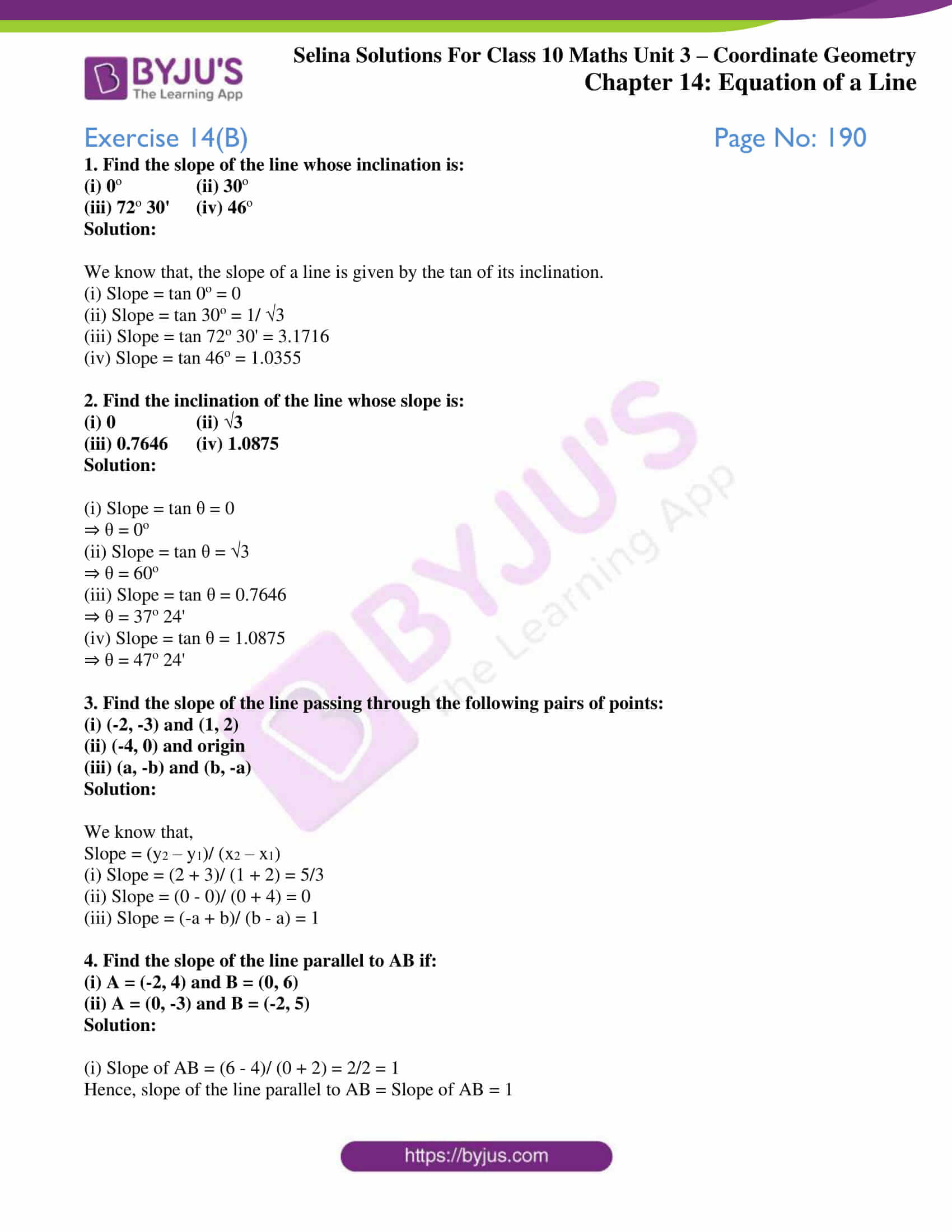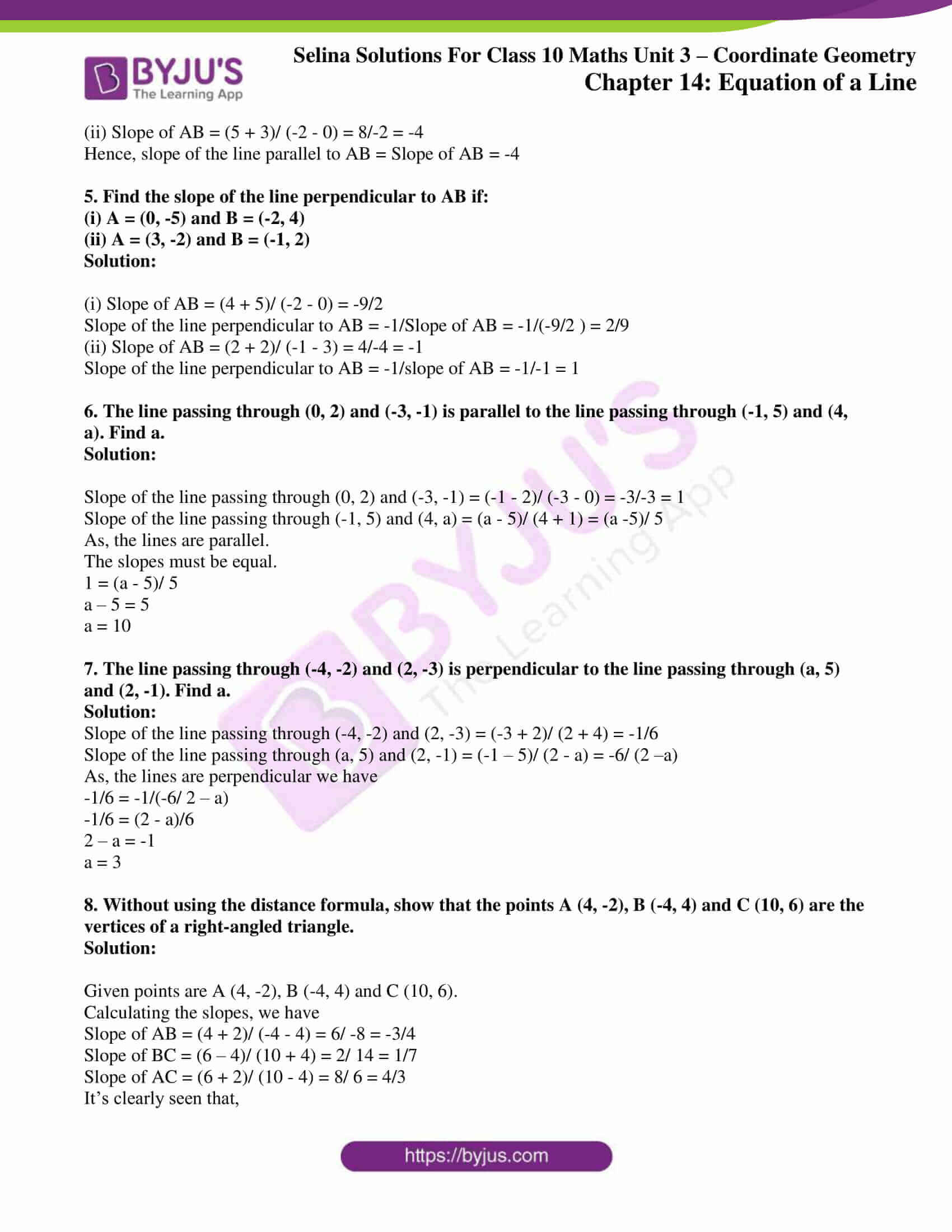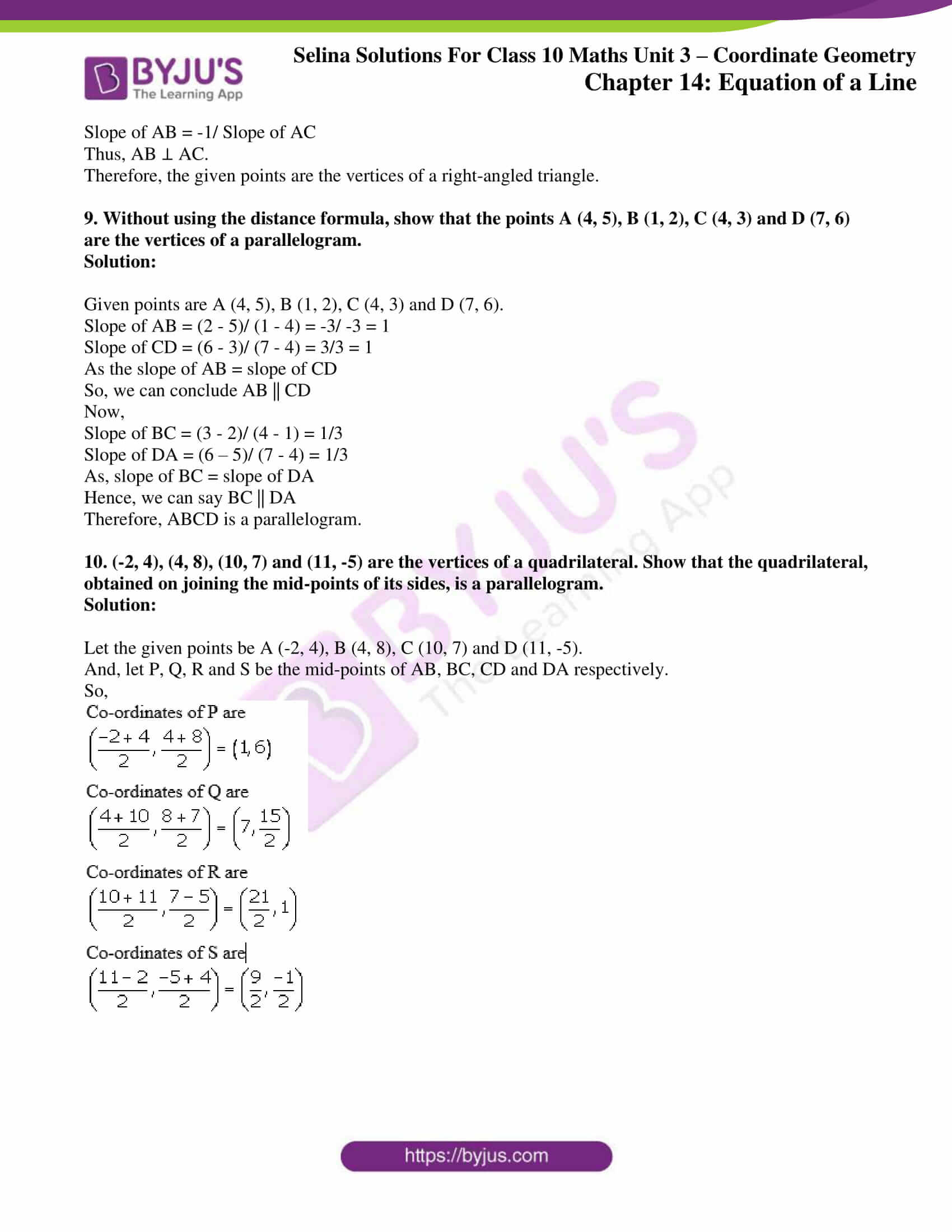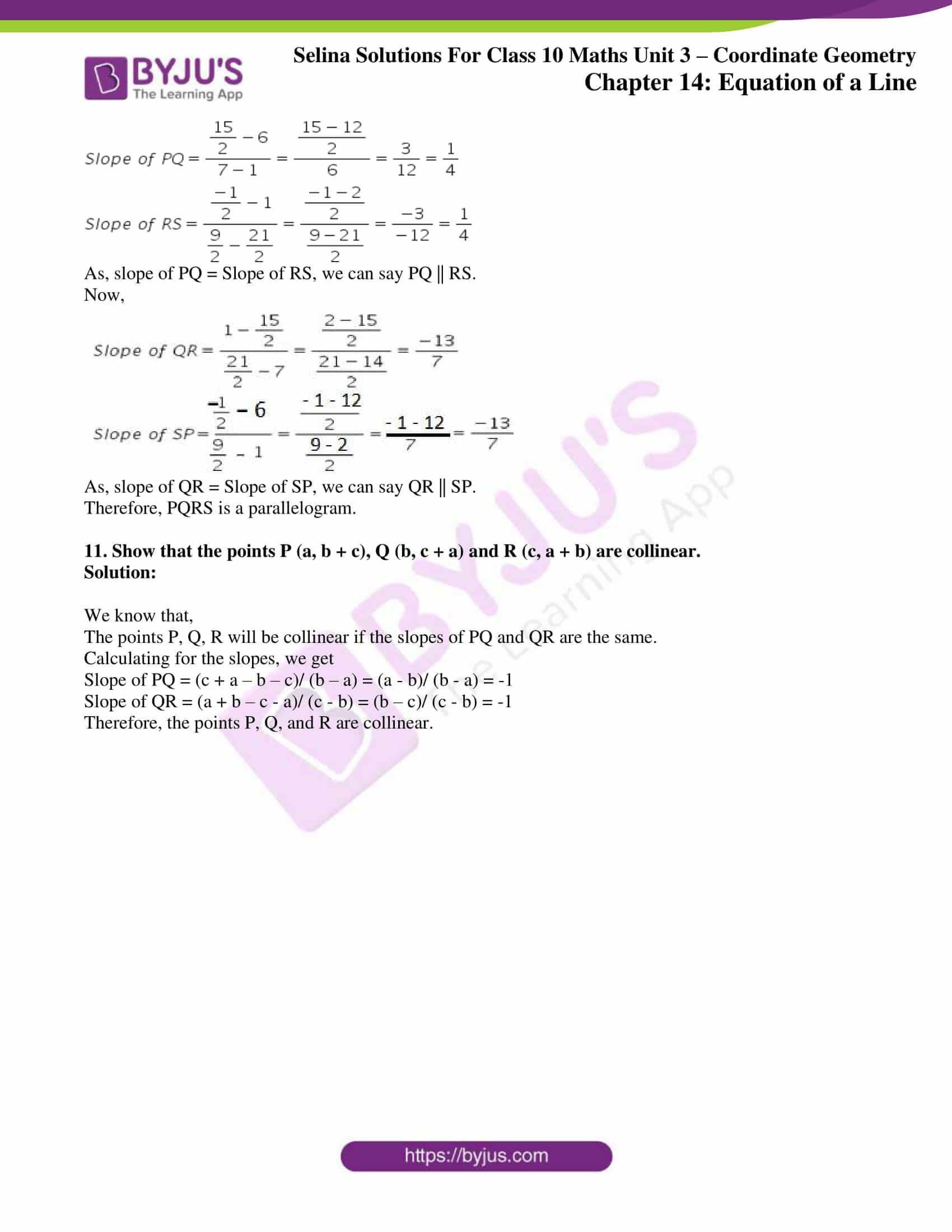### Access other exercises of Selina Solutions Concise Maths Class 10 Chapter 14 Equation of a Line

Exercise 14(A) Solutions

Exercise 14(C) Solutions

Exercise 14(D) Solutions

Exercise 14(E) Solutions

### Access Selina Solutions Concise Maths Class 10 Chapter 14 Equation of a Line Exercise 14(B)

#### Exercise 14(B) Page No: 190

1. Find the slope of the line whose inclination is:

(i) 0o  (ii) 30o

(iii) 72o 30′ (iv) 46o

Solution:

We know that, the slope of a line is given by the tan of its inclination.

(i) Slope = tan 0o = 0

(ii) Slope = tan 30o = 1/ √3

(iii) Slope = tan 72o 30′ = 3.1716

(iv) Slope = tan 46o = 1.0355

2. Find the inclination of the line whose slope is:

(i) 0 (ii) √3

(iii) 0.7646 (iv) 1.0875

Solution:

(i) Slope = tan θ = 0

⇒ θ = 0o

(ii) Slope = tan θ = √3

⇒ θ = 60o

(iii) Slope = tan θ = 0.7646

⇒ θ = 37o 24′

(iv) Slope = tan θ = 1.0875

⇒ θ = 47o 24′

3. Find the slope of the line passing through the following pairs of points:

(i) (-2, -3) and (1, 2)

(ii) (-4, 0) and origin

(iii) (a, -b) and (b, -a)

Solution:

We know that,

Slope = (y2 – y1)/ (x2 – x1)

(i) Slope = (2 + 3)/ (1 + 2) = 5/3

(ii) Slope = (0 – 0)/ (0 + 4) = 0

(iii) Slope = (-a + b)/ (b – a) = 1

4. Find the slope of the line parallel to AB if:

(i) A = (-2, 4) and B = (0, 6)

(ii) A = (0, -3) and B = (-2, 5)

Solution:

(i) Slope of AB = (6 – 4)/ (0 + 2) = 2/2 = 1

Hence, slope of the line parallel to AB = Slope of AB = 1

(ii) Slope of AB = (5 + 3)/ (-2 – 0) = 8/-2 = -4

Hence, slope of the line parallel to AB = Slope of AB = -4

5. Find the slope of the line perpendicular to AB if:

(i) A = (0, -5) and B = (-2, 4)

(ii) A = (3, -2) and B = (-1, 2)

Solution:

(i) Slope of AB = (4 + 5)/ (-2 – 0) = -9/2

Slope of the line perpendicular to AB = -1/Slope of AB = -1/(-9/2 ) = 2/9

(ii) Slope of AB = (2 + 2)/ (-1 – 3) = 4/-4 = -1

Slope of the line perpendicular to AB = -1/slope of AB = -1/-1 = 1

6. The line passing through (0, 2) and (-3, -1) is parallel to the line passing through (-1, 5) and (4, a). Find a.

Solution:

Slope of the line passing through (0, 2) and (-3, -1) = (-1 – 2)/ (-3 – 0) = -3/-3 = 1

Slope of the line passing through (-1, 5) and (4, a) = (a – 5)/ (4 + 1) = (a -5)/ 5

As, the lines are parallel.

The slopes must be equal.

1 = (a – 5)/ 5

a – 5 = 5

a = 10

7. The line passing through (-4, -2) and (2, -3) is perpendicular to the line passing through (a, 5) and (2, -1). Find a.

Solution:

Slope of the line passing through (-4, -2) and (2, -3) = (-3 + 2)/ (2 + 4) = -1/6

Slope of the line passing through (a, 5) and (2, -1) = (-1 – 5)/ (2 – a) = -6/ (2 –a)

As, the lines are perpendicular we have

-1/6 = -1/(-6/ 2 – a)

-1/6 = (2 – a)/6

2 – a = -1

a = 3

8. Without using the distance formula, show that the points A (4, -2), B (-4, 4) and C (10, 6) are the vertices of a right-angled triangle.

Solution:

Given points are A (4, -2), B (-4, 4) and C (10, 6).

Calculating the slopes, we have

Slope of AB = (4 + 2)/ (-4 – 4) = 6/ -8 = -3/4

Slope of BC = (6 – 4)/ (10 + 4) = 2/ 14 = 1/7

Slope of AC = (6 + 2)/ (10 – 4) = 8/ 6 = 4/3

It’s clearly seen that,

Slope of AB = -1/ Slope of AC

Thus, AB ⊥ AC.

Therefore, the given points are the vertices of a right-angled triangle.

9. Without using the distance formula, show that the points A (4, 5), B (1, 2), C (4, 3) and D (7, 6) are the vertices of a parallelogram.

Solution:

Given points are A (4, 5), B (1, 2), C (4, 3) and D (7, 6).

Slope of AB = (2 – 5)/ (1 – 4) = -3/ -3 = 1

Slope of CD = (6 – 3)/ (7 – 4) = 3/3 = 1

As the slope of AB = slope of CD

So, we can conclude AB || CD

Now,

Slope of BC = (3 – 2)/ (4 – 1) = 1/3

Slope of DA = (6 – 5)/ (7 – 4) = 1/3

As, slope of BC = slope of DA

Hence, we can say BC || DA

Therefore, ABCD is a parallelogram.

10. (-2, 4), (4, 8), (10, 7) and (11, -5) are the vertices of a quadrilateral. Show that the quadrilateral, obtained on joining the mid-points of its sides, is a parallelogram.

Solution:

Let the given points be A (-2, 4), B (4, 8), C (10, 7) and D (11, -5).

And, let P, Q, R and S be the mid-points of AB, BC, CD and DA respectively.

So,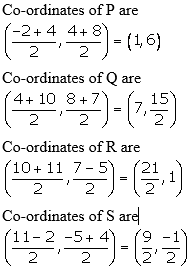As, slope of PQ = Slope of RS, we can say PQ || RS.

Now,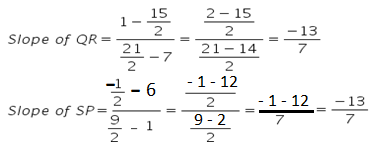As, slope of QR = Slope of SP, we can say QR || SP.

Therefore, PQRS is a parallelogram.

11. Show that the points P (a, b + c), Q (b, c + a) and R (c, a + b) are collinear.

Solution:

We know that,

The points P, Q, R will be collinear if the slopes of PQ and QR are the same.

Calculating for the slopes, we get

Slope of PQ = (c + a – b – c)/ (b – a) = (a – b)/ (b – a) = -1

Slope of QR = (a + b – c – a)/ (c – b) = (b – c)/ (c – b) = -1

Therefore, the points P, Q, and R are collinear.